## Table of ContentOpen Access

ARTICLE

# Throughput Analysis of HARQ Scheme Based on Full-Duplex Two-Way AF SWIPT Relay

Xiaoye Shi1,*, Fei Ding1,2, Haiting Zhu1, Zhaowei Zhang1, Lei Zhang1

1 School of Internet of Things (Nanjing University of Posts and Telecommunications), Nanjing, 210003, China
2 Department of Electronic and Electrical Engineering (University College London), London, WC1E6BT, UK

* Corresponding Author: Xiaoye Shi. Email:Computers, Materials & Continua 2023, 74(3), 4819-4830. https://doi.org/10.32604/cmc.2023.018637

## Abstract

The simultaneous wireless information and power transfer (SWIPT) relay system is one of the emerging technologies. Xiaomi Corporation and Motorola Inc. recently launched indoor wireless power transfer equipment is one of the most promising applications. To tap the potential of the system, hybrid automatic repeat request (HARQ) is introduced into the SWIPT relay system. Firstly, the time slot structure of HARQ scheme based on full duplex two-way amplify and forward (AF) SWIPT relay is given, and its retransmission status is analyzed. Secondly, the equivalent signal-to-noise ratio and outage probability of various states are calculated by approximate simplification. Thirdly, the energy harvesting power in each state is calculated. Finally, the energy harvested-throughput sum function is constructed to characterize the performance of energy harvesting and data transmission. Simulation results show that the proposed HARQ scheme has better energy harvested-throughput sum function than the traditional HARQ scheme. When P2 = 22 dB, the maximum sum function is 54.86% (the proposed HARQ scheme) and 52.307% (the traditional HARQ scheme), respectively.

## Keywords

1  Introduction

With the realization of wireless power transfer technology of cellular network  and sensor networks [4,5], new researches upsurge appear. Because it is not limited to the battery capacity, the simultaneous wireless information and power transfer (SWITP) technology makes the battery's super long endurance becomes a reality . Xiaomi Corporation and Motorola Inc. recently launched indoor wireless power transfer equipment is one of the most promising applications. By amplifying and forwarding the received signal, the relay can not only help the information transmission, but also promote the wireless power transfer [7,8]. Two-way full-duplex relay can further improve the information transmission efficiency of the SWIPT relay system, with providing more flexible transmission strategy [9,10].

The hybrid automatic repeat request (HARQ) technology improve the reliability of data transmission through error detection and data retransmission . Furthermore, HARQ improve the effectiveness of the system by the maximum ratio combining of the data received [12,13]. In , the HARQ technology was applied in two-way communication. The research show that the transmission capacity obtained by full duplex transmission and packet retransmission through HARQ protocol exceeds the loss caused by bidirectional use and HARQ. In order to improve the throughput of the system, full duplex relay was used in , and a reverse link assisted network coding HARQ with indirect information was used at the relay port. Reference  studied the performance of full duplex cooperative networks under HARQ from two aspects of data link layer packet error rate (per) and master-slave throughput. The cooperative relay scheme without initialization phase is proposed to realize HARQ retransmission assisted by complex relay .

How to combine HARQ technology with SWIPT technology to improve the efficiency of data and energy transmission is a fascinating novel subject. Reference  propose the optimal strategy aiming at the minimum expected retransmission times and accumulate mutual information. Unfortunately, for SWIPT systems, accumulate mutual information are not comprehensive. This paper mainly studies the application of energy carrying relay and HARQ in full duplex two-way amplify and forward (AF) relay system. According to the characteristics of full-duplex two-way AF relay and energy carrying relay, a HARQ scheme based on full-duplex two-way AF relay is proposed, and its performance is analyzed. Based on this, the following work is done: a) The slot structure of HARQ scheme based on full duplex bidirectional AF energy carrying relay is given, and its retransmission status is analyzed. b) The equivalent signal-to-noise ratio and outage probability of various states are calculated by approximate simplification. c) The power of energy harvesting in each state is calculated. d) The sum function of throughput-energy harvesting is constructed, and the performance of data transmission and energy harvesting is characterized.

2  System Model of the Full-Duplex Two-Way SWIPT Relay System

Fig. 1 is the system diagram of full-duplex two-way SWIPT relay system, which is composed of two terminals T1, T2 and relay node R. The system consists of four communication links including direct link between T1 and T2 and two swift links, in which energy is transmitted from relay node to two terminals. In this system, the relay node R adopts the AF mode, and also takes into account the role of energy transmission. Terminal nodes T1 and T2 adopt full duplex mode, that is, nodes can also send data at the same time when receiving data, so as to obtain transmission rate more efficiently, but also cause self-interference problem. The access mode of the system is time division multiple access (TDMA).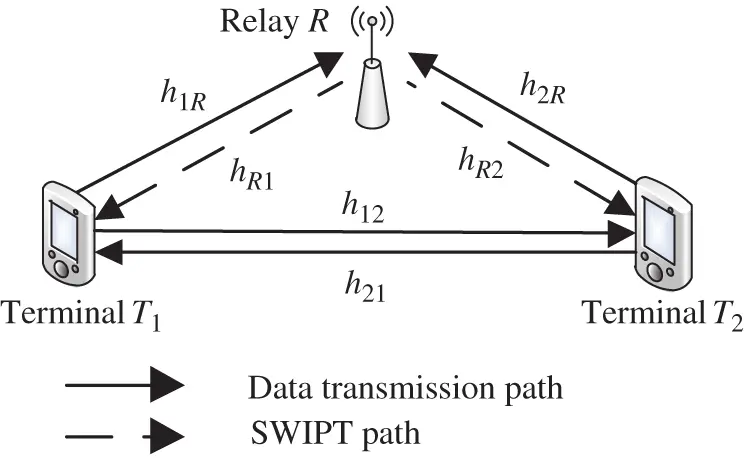Figure 1: System model of the full-duplex two-way SWIPT relay system

hab(a{1,R,2},b{1,R,2}) is used to represent the channel gain of each channel, which follows the complex Gaussian distribution with mean value of 0 and variance of σab2(a{1,R,2},b{1,R,2}) (where h11 and h22 are the channel gain of self-interference channel); nabc(a{1,R,2},b{1,R,2}) is used to represent the circuit noise caused by energy harvesting, which is subject to complex Gaussian distribution with mean value of 0 and variance of Nabc(a{1,R,2},b{1,R,2}); nabe(a{1,R,2},b{1,R,2}) is used to represent the equivalent noise caused by signal demodulation, which obeys the complex Gaussian distribution with mean value of 0 and variance of Nabe(a{1,R,2},b{1,R,2}). The total transmission power of the system is PT, and the power allocated to two terminals T1, T2 and relay R is P1, P2 and PR, i.e., P1+P2+PR=PT. Assuming that the uplink channel and downlink channel are symmetric channels, then hab=hba(a{1,R,2},b{1,R,2}).

3  System Slot Structure of the Full-Duplex Two-Way SWIPT Relay System

As shown in Tab. 1, the system is divided into two time slots. In the first time slot, data are transmitted and received by two terminals T1 and T2 at the same time, and the relay node receives the mixed signal of the two terminals. Terminal T1 and T2 detect the received signal cyclic redundancy check (CRC) to determine whether the system needs retransmission. The second time slot is the SWIPT time slot, and the relay node sends the retransmission data and energy simultaneously. The second time slot is divided into four states: when two terminals in one time slot receive data successfully, the relay node only transmits energy; when both terminals in the first slot fail to receive data successfully, the relay node mainly transmits data, supplemented by energy transmission; when only one terminal in the first slot receives data successfully, the terminal receiving data successfully mainly transmits energy and another terminal is mainly for data transmission.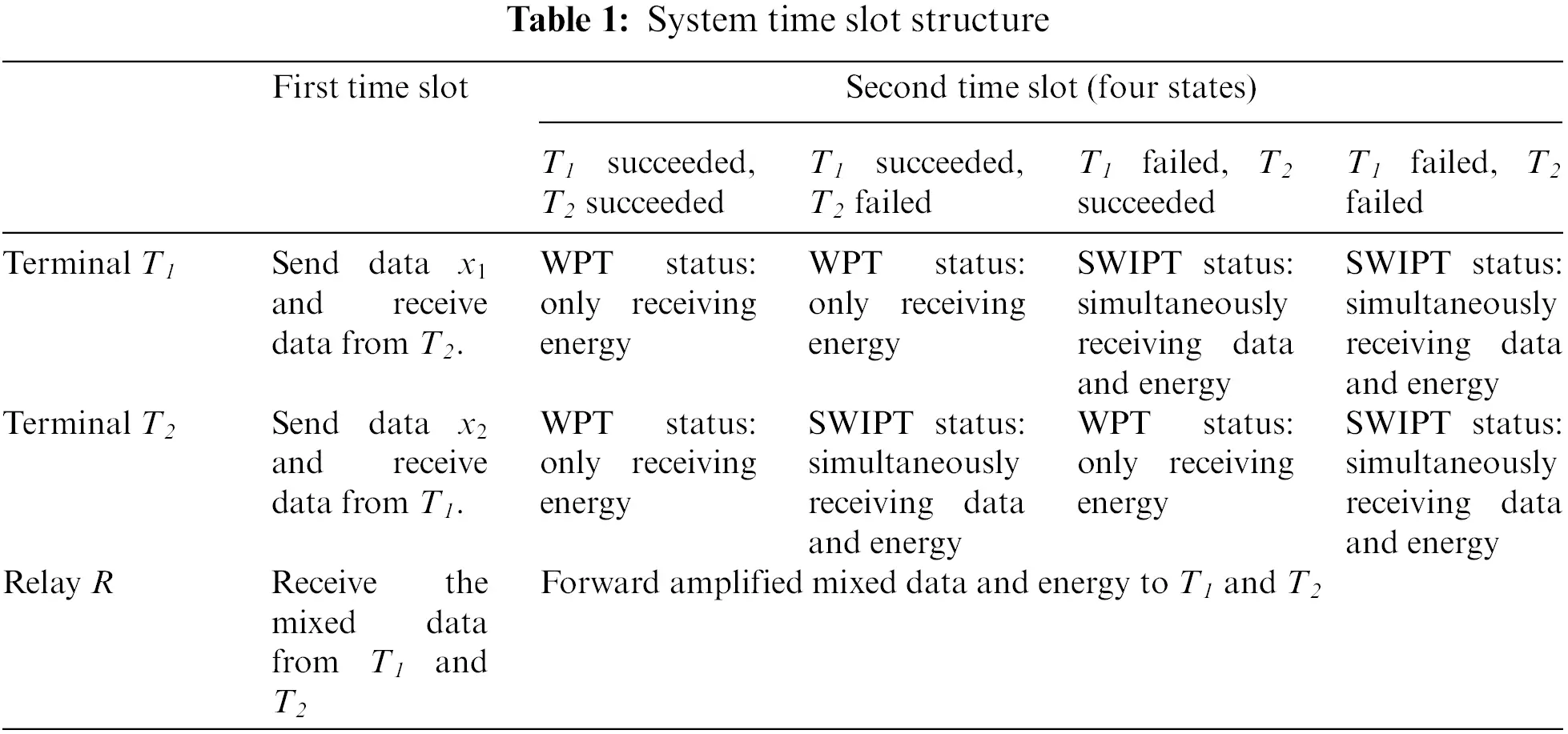with x1 and x2 are the data to be transmitted by terminals T1 and T2 respectively.

In the first time slot, two terminals T1 and T2 transmit and receive data at the same time. The relay node receives the mixed signal of the two terminals, so the received signals of the three nodes are as follows:

y12=P1(h12x1)+P2h22x2+n12e,(1)

y21=P2(h21x2)+P1h11x1+n21e,(2)

yR=P1(h1Rx1)+P2(h2Rx2)+nR,(3)

where nR represents the noise signal received by the first time slot relay node, which obeys the complex Gaussian distribution with mean value of 0 and variance of NR. P1(h12x1) and P2(h21x2) are the effective signals received by two terminals T1 and T2 respectively. P2h22x2 and P2(h11x2) are self-interference signals. Therefore, the received signal-to-noise ratio (SNR) of two terminals T1 and T2 in the first time slot is SNR21=P2|h21|2P1|h11|2+N21e and SNR12=P1|h12|2P2|h22|2+N12e respectively.

The outage probability of T1 and T2 signals in the first slot is as follows:

Pout12=Pr{SNR12<22R1}=1exp(1σ12222R1θ1,1),(4)

Pout21=Pr{SNR21<22R1}=1exp(1σ21222R1θ2,1),(5)

where θ1,1=P2P1σ112+N21e, θ2,1=P1P2σ222+N12e.

In the second time slot, the relay node sends retransmission data and energy at the same time. At this moment, the received signals of the two terminals T1 and T2 can be expressed as follows:

yR1=G(hR1yR)+nR1e,(6)

yR2=G(hR2yR)+nR2e,(7)

where the amplification factor is G=PRP1|h1R|2+P2|h2R|2+nR.

If the two terminals are in the state of SWIPT in the second slot, the received information data are as follows:

yR1c=τ(GhR1h2RP2x2+GhR1nR+nR1e)+nR1c,(8)

yR2c=τ(GhR2h1RP1x1+GhR2nR+nR2e)+nR2c,(9)

with τ is the proportion of energy allocated to information reception, and the rest 1τ is used for energy harvesting.

At this time, the received signal-to-noise ratio of terminal T1 is:

SNRR1c=τPRP2|hR1|2|h2R|2τPR|hR1|2NR+(τNR2e+NR2c)(P1|h1R|2+P2|h2R|2+NR)=θ1,5|hR1|2|h2R|2θ1,2|hR1|2+θ1,3|h2R|2+θ1,4=θ1,2θ1,3θ1,42|hR1|2|h2R|2θ1,2θ1,4|hR1|2+θ1,3θ1,4|h2R|2+1θ1,5θ1,4θ1,2θ1,3,(10)

where θ1,2=(τPRNR+(τNR2e+NR2c)P1), θ1,3=P2(τNR2e+NR2c), θ1,4=NR(τNR2e+NR2c), θ1,5=τPRP2.

Similarly, the receiving signal-to-noise ratio (SNR) of terminal T2 is as follows:

SNRR2c=θ2,2θ2,3θ2,42|hR2|2|h1R|2θ2,2θ2,4|hR2|2+θ2,3θ2,4|h1R|2+1θ2,5θ2,4θ2,2θ2,3,(11)

where θ2,2=(τPRNR+(τNR1e+NR1c)P2), θ2,3=P1(τNR1e+NR1c), θ2,4=NR(τNR1e+NR1c), θ2,5=τPRP1.

4  System Throughput and Sum Function of the Full-Duplex Two-Way SWIPT Relay System

In order to briefly describe the receiving process, the following scenario of T2 transmission and T1 reception is taken as an example:

When the CRC detection of the first slot is successful, the power divider allocates all the energy to the energy harvesting, that is τ=0, the terminal is in WPT state at the moment. The power of energy harvesting is as follows:

E1P=ησR12PR,(12)

with η is the conversion efficiency of energy harvesting.

If the CRC detection of the first time slot fails, the terminal is in the SWIPT state. T1 combines the signals about x2 received by the two time slots with the maximum ratio, and the combined equivalent signal-to-noise ratio is the sum of twice received SNRs:

SNRARQ,1=SNR21+SNRR1c=|h21|2θ1,1+θ1,2θ1,3θ1,42|hR1|2|h2R|2θ1,2θ1,4|hR1|2+θ1,3θ1,4|h2R|2+1θ1,5θ1,4θ1,2θ1,3=(|h21|2θ1,1θ1,2θ1,3θ1,4θ1,5+θ1,2θ1,3θ1,42|hR1|2|h2R|2θ1,2θ1,4|hR1|2+θ1,3θ1,4|h2R|2+1)θ1,5θ1,4θ1,2θ1,3.(13)

Outage probability PoutARQ,1 is:

Pr{SNRARQ,1<22R1}=Pr{(|h21|2θ1,1θ1,2θ1,3θ1,4θ1,5+θ1,2θ1,3θ1,42|hR1|2|h2R|2θ1,2θ1,4|hR1|2+θ1,3θ1,4|h2R|2+1)<(22R1)θ1,2θ1,3θ1,4θ1,5}12σ212θ1,1θ1,2θ1,3θ1,4θ1,5(θ1,2θ1,4σR12+θ1,3θ1,4σR22θ1,2θ1,4σR12θ1,3θ1,4σR22)((22R1)θ1,2θ1,3θ1,4θ1,5)2=12σ212θ1,1θ1,5(θ1,2σR12+θ1,3σR22σR12σR22)((22R1))2.(14)

After combining the received signals of two time slots, the outage probability of terminal T1 is as follows:

Poutt,1=1(1Pout21+Pout21Pr((SNRARQ,122R1)|(SNR21<22R1)))=1(1Pout21+Pout21Pr(SNR21<22R1)Pr(SNRARQ,1<22R1,SNR21<22R1)Pr(SNR21<22R1))=1(1Pout21+Pout21(1PoutARQ,1Pout21))=PoutARQ,1.(15)

The outage probability of T1 received signal is the same as that the traditional HARQ scheme. Similarly, we may get the outage probability Poutt,2 of T2 signal (The expression is omitted).

The unification throughput of terminals T1 and T2 is TP1=1Poutt,1, TP2=1Poutt,2. The total unification throughput of the system is:

TPT=TP1+TP22.(16)

The second slot, if T1 is in SWIPT state, the energy that can be harvested is as follows:

E1S=(1τ)ησR12PR.(17)

Therefore, the total energy harvested by T1 is

E1T=Pout21E1S+(1Pout21)E1P.(18)

By introducing Pout21=1σ21222R1θ1,1 into the above formula, the following conclusions can be obtained:

E1T=1σ21222R1θ1,1E1S+(11σ21222R1θ1,1)E1P=ησR12PR+1σ21222R1θ1,1((1τ)ησR12PRησR12PR)=ησR12PR(11σ21222R1θ1,1τ).(19)

Similarly, the power of the energy harvested by terminal T2 is easy to be obtained (The expression is omitted).

To sum up, the total average power of energy harvested by the system is:

ET=E1T+E2T2.(20)

In order to describe the performance of the proposed HARQ scheme more comprehensively, the sum function of system throughput and energy acquisition power is constructed:

=TPT+ET=PoutARQ,1+PoutARQ,22+ησR12PR(11σ21222R1θ1,1τ)+ησR22PR(11σ12222R1θ2,1τ)2.(21)

5  Simulation Analysis

In this section, the correctness of the above analysis is verified by computer simulation. Suppose the variance N21e, N12e, NR1e and NR2e of signal demodulation noise is 1, the variance of circuit noise is NR1c=0.1, NR2c=0.1, energy collection efficiency η is 0.8, the energy ratio of information receiving τ is 0.5, and the information rate threshold R is 1 (i.e., the signal-to-noise ratio satisfies SNR12<2211).

The outage probability of full duplex direct link versus power P2 is presented in Fig. 2. In Fig. 2, the abscissa is the transmitting power P2 of terminal T2 in the first time slot, and the ordinate is the outage probability of the signal transmitted by terminal T2 in the first slot. The parameters are set as follows: the total power of the system is PT = 20 dBW, P1 = 0.1 P, PR = PT P1 P2, the gain of transmission channel is σ122=1, σr12=1 and σr22=1, the variance of self-interference is σ222=0.1. “∆” is the Outage probability curve calculated theoretically and “+” is the simulation curve. It is obvious that the theoretical curve coincides with the simulation curve.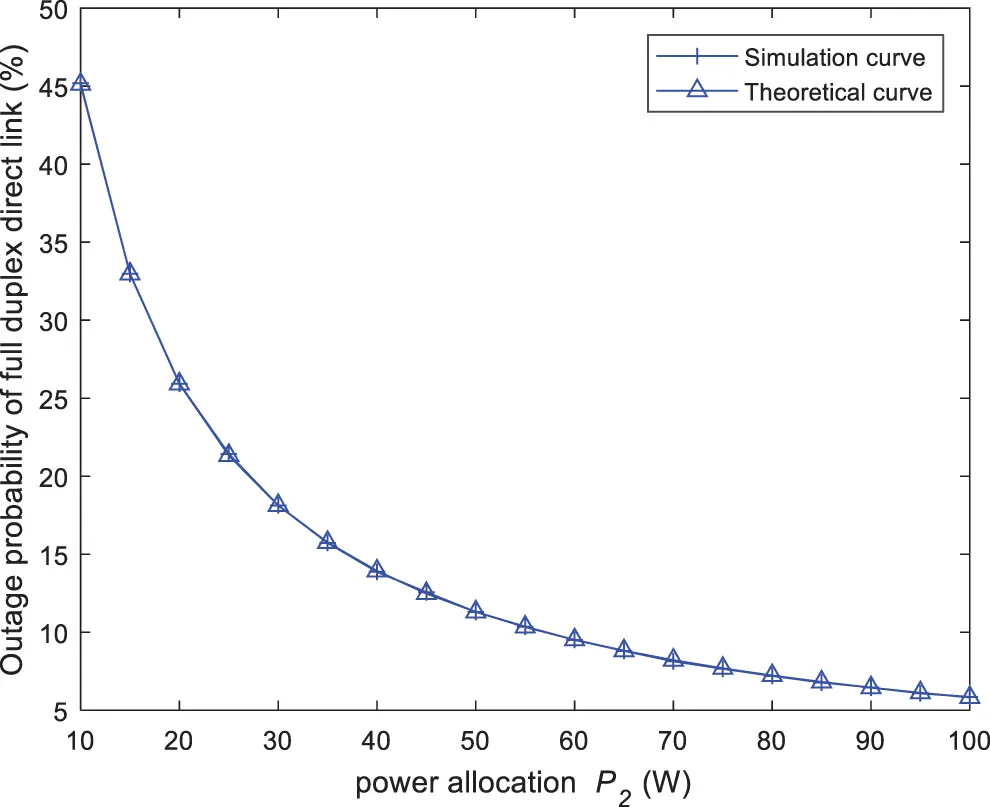Figure 2: The outage probability of full duplex direct link vs. power P2

For better insights, the throughput of terminal T2 against power P2 is given in Fig. 3. In Fig. 3, the abscissa is the transmitting power P2 of terminal T2 in the first time slot, the ordinate is the total throughput of terminal T2 in the two time slots. “∆” is the throughput curve calculated theoretically. The parameters are the same as those in Fig. 2. When P2 is small, the throughput of terminal T2 increases with the increase of P2. This is because the transmission power of the first slot determines the outage probability of the direct link as shown in Fig. 2. However, when P2 increases to a certain extent, the transmission power of the relay is severely limited due to the limitation of the total power, which leads to a decrease in the total throughput. Therefore, reasonable power allocation may improve the performance of total throughput.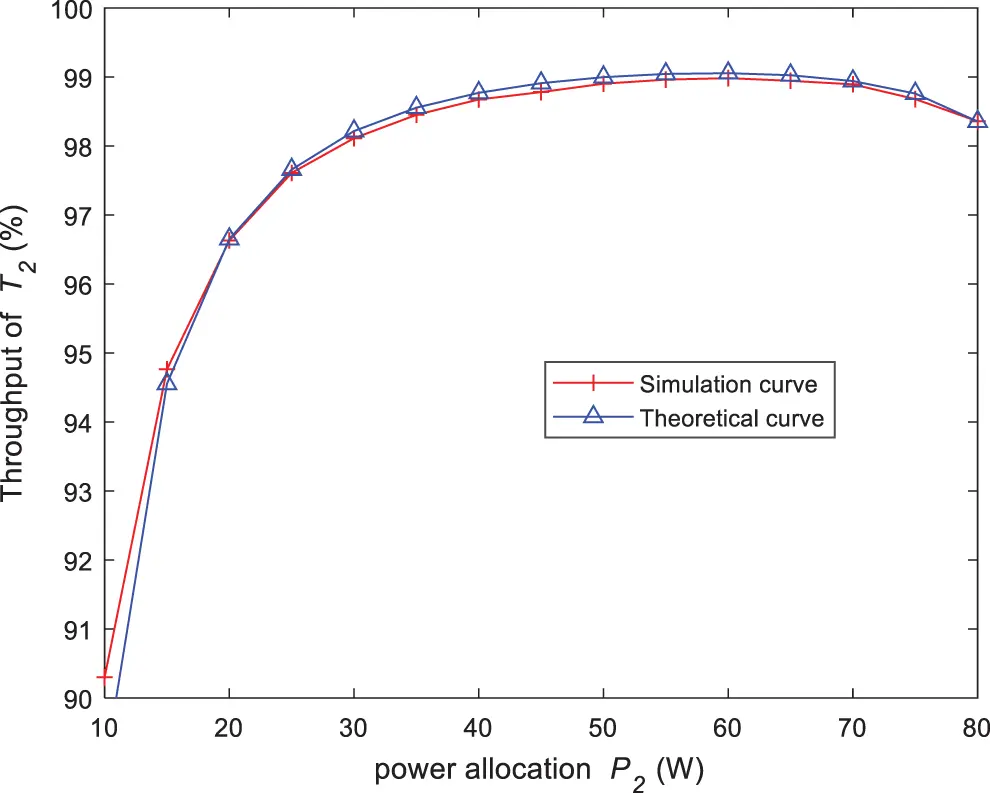Figure 3: The throughput of terminal T2 against power P2

As shown in Fig. 4, the abscissa is the transmission power P1, and the ordinate is the total throughput of terminal T2 in two time slots. The total system power is PT = 30 dBW, P2 = 0.1 P, PR = PT P1 P2. Other parameters are the same as those set in Fig. 2. With the increase of P1, the power used to transmit data of T2 decreases, especially the forwarding power at the relay. Obviously, the total throughput of terminal T2 gradually decreases with the increase of P1.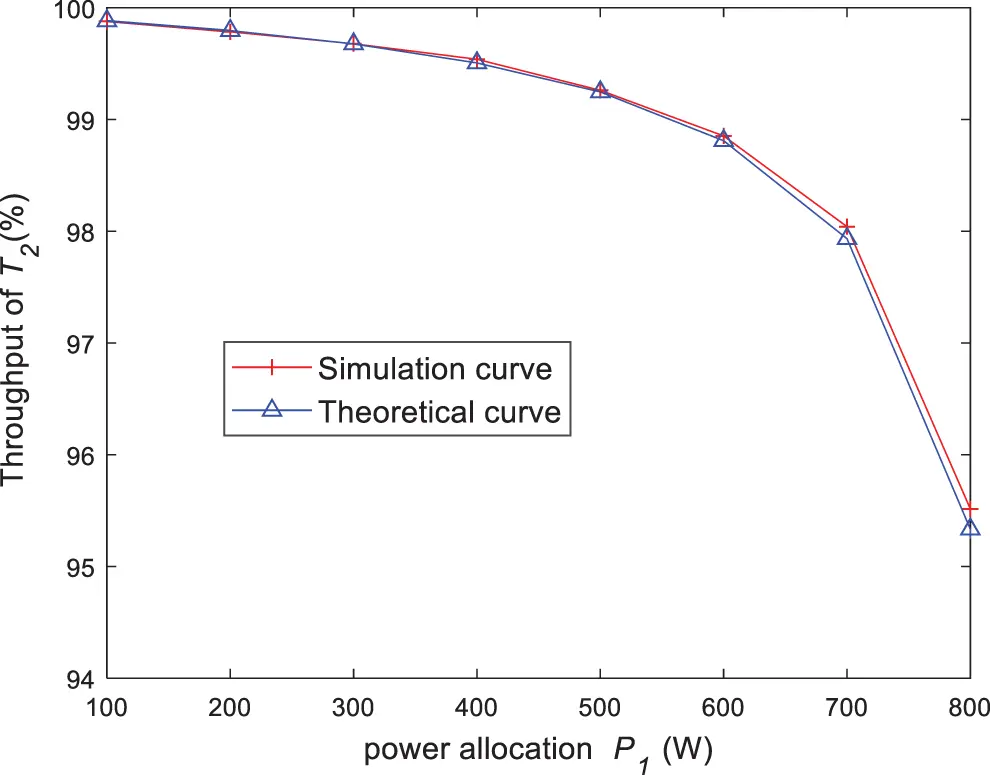Figure 4: The throughput of terminal T2 against power P1

The relationship between the total throughput of the system and power of the terminal T2 under the symmetrical channel is indicated in Fig. 5. The parameters in Fig. 5 are the same as those set in Fig. 4, except for PT = 30 dBW, PR = 0.1 P and P1 = PT PR P2. Because the total throughput of the system is the sum of the throughput of two terminals, little transmission power of terminal T1 and terminal T2 may affect the total throughput. As shown in Fig. 5, the total throughput of the system is a symmetric curve. The channel between the two terminals and the relay in Fig. 5 is symmetrical channel, so the total system throughput reaches the maximum when the two terminals power equals to each other.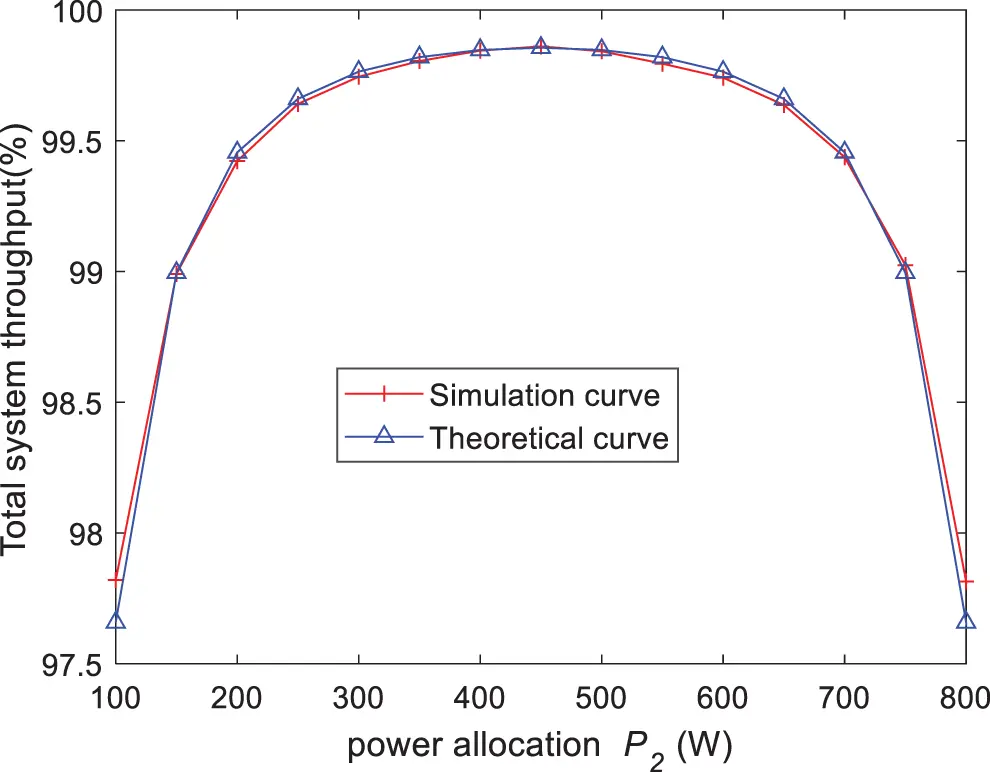Figure 5: The relationship between the total throughput of the system and power of the terminal T2 under the symmetrical channel

The total system throughput against P2 is illustrated in Fig. 6 under asymmetric channel. The parameter is σr12=4 in Fig. 6. In this case, the channel quality from R to terminal T1 is better than that from R to terminal T2. In order to achieve the same throughput of two terminals, it is necessary to allocate more power to terminal T2. As shown in Fig. 6, when the total throughput of the system reaches maximum, the power allocated to terminal T2 (400 W) is close to and less than the average allocation (450 W).Figure 6: The total system throughput against P2 under the asymmetric channel

Fig. 7 compares the energy harvested-throughput sum function of the two schemes in asymmetric channels (the proposed HARQ scheme and the traditional HARQ scheme). Except for PT = 25 dBW, other parameters are the same as those in Fig. 6. It is obvious that the sum function of the proposed HARQ scheme is better than that of the traditional HARQ scheme. When P2 = 22 dB, the maximum sum function is 54.86% (the proposed HARQ scheme) and 52.307% (the traditional HARQ scheme), respectively.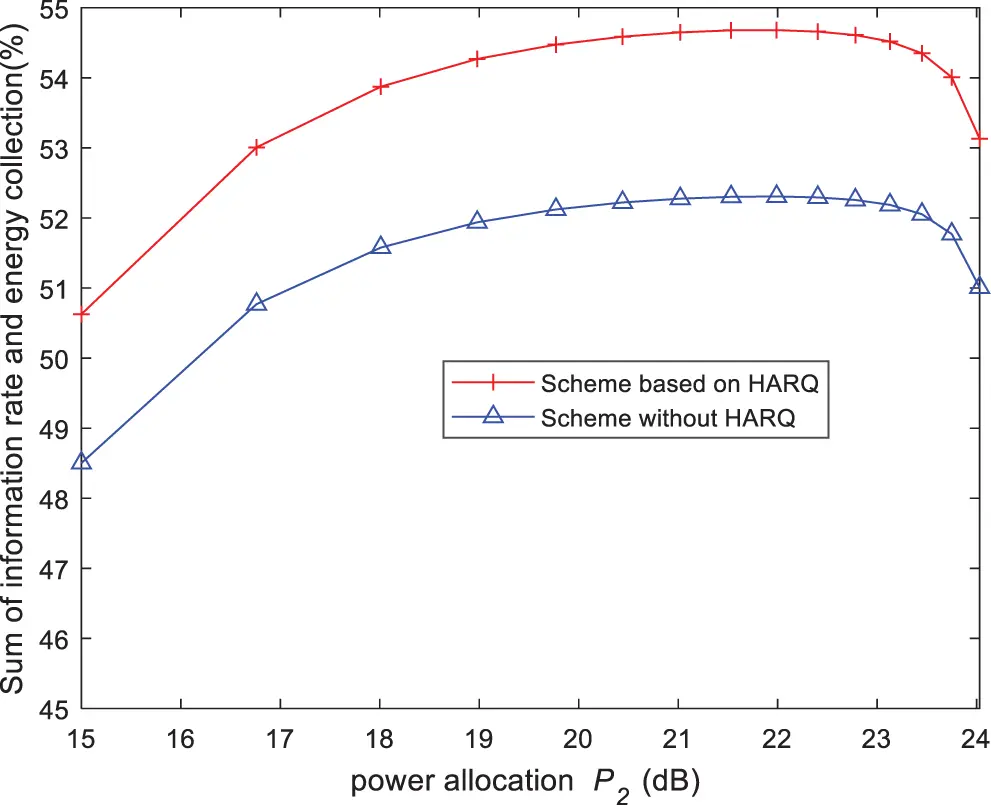Figure 7: The energy harvested-throughput sum function of the two schemes in asymmetric channels

6  Conclusions

In order to further explore the potential of the SWIPT relay system, the full duplex two-way SWIPT relay HARQ scheme is proposed in this paper. Firstly, the received signal-to-noise ratio (SNR) is calculated by analyzing the system model and time slot structure. Then the outage probability and throughput are obtained by approximate simplification. Then, the energy harvested power in various states is obtained. Finally, the energy harvested-throughput sum function are constructed. Simulation results show that the proposed HARQ scheme has better energy harvested-throughput sum function than the traditional HARQ scheme.

Acknowledgement: The authors would like to thank the anonymous reviewers for their constructive comments and suggestions.

Funding Statement: This work was supported by the National Natural Science Foundation of China (Grants No. 61701251, 62071244, 62071249, 61872423, 61801236 and 61806100), Open Fund of Key Laboratory of Icing and Anti/De-icing (Grant No. IADL20190105) and the Natural Science Foundation of Jiangsu Province (Grants No. BK20160903).

Conflicts of Interest: The authors declare that they have no conflicts of interest to report regarding the present study.

## References

1. Al-Baidhani, M. Vehkapera and M. Benaissa, “Simultaneous wireless information and power transfer based on generalized triangular decomposition,” IEEE Transactions on Green Communications and Networking, vol. 3, no. 3, pp. 751–764, 2019.
2. T. A. Le, Q. Vien, H. X. Nguyen, D. W. K. Ng and R. Schober, “Robust chance-constrained optimization for power-efficient and secure SWIPT systems,” IEEE Transactions on Green Communications and Networking, vol. 1, no. 3, pp. 333–346, 2017.
3. Y. Ji, W. Duan, M. Wen, P. Padidar and P. Ho, “Spectral efficiency enhanced cooperative device-to-device systems with NOMA,” IEEE Transactions on Intelligent Transposition Systems, vol. 99, pp. 1–11, 2020.
4. H. Zhu, D. Gao and S. Zhang, “A perceptron algorithm for forest fire prediction based on wireless sensor networks,” Journal of Internet of Things, vol. 1, no. 1, pp. 25–31, 2019.
5. M. Okhovvat and M. R. Kangavari, “Tslbs: A time-sensitive and load balanced scheduling approach to wireless sensor actor networks,” Computer Systems Science and Engineering, vol. 34, no. 1, pp. 13–21, 2019.
6. S. Kaur and V. K. Joshi, “Hybrid soft computing technique based trust evaluation protocol for wireless sensor networks,” Intelligent Automation & Soft Computing, vol. 26, no. 2, pp. 217–226, 2020.
7. M. K. Shukla, H. H. Nguyen and O. J. Pandey, “Multiuser full-duplex IoT networks with wireless-powered relaying: Performance analysis and energy efficiency optimization,” IEEE Transactions on Green Communications and Networking, vol. 4, no. 4, pp. 982–997, 2020.
8. Y. Yan and S. Zhang, “Energy efficiency maximization of the SWIPT-based full-duplex relay cooperative system,” in Proc. IEEE Int. Conf. on Communication Technology, Xi’an, China, pp. 398–403, 2019.
9. X. Shi, J. Sun, D. Li, F. Ding and Z. Zhang, “Rate-energy tradeoff for wireless simultaneous information and power transfer in full-duplex and half-duplex systems,” Computer, Materials and Continua, vol. 65, no. 2, pp. 1373–1384, 2020.
10. X. Pei, W. Duan, M. Wen, Y. Wu and V. Monteiro, “Socially-aware joint resource allocation and computation offloading in NOMA-aided energy harvesting massive IoT,” IEEE Internet of Things Journal, vol. 8, no. 7, pp. 5240–5249, 2021.
11. K. Xu, Y. Gao, Y. Xu and W. Yang, “Diversity-multiplexing tradeoff analysis of AF two-way relaying channel with hybrid ARQ over rayleigh fading channels,” IEEE Transactions on Vehicular Technology, vol. 63, no. 3, pp. 1504–1510, 2014.
12. S. Nam, M. Alouini and S. Choi, “Iterative relay scheduling with hybrid ARQ under multiple user equipment (type II) relay environments,” IEEE Access, vol. 6, pp. 6455–6463, 2018.
13. J. Su, R. Xu, S. Yu, B. Wang and J. Wang, “Redundant rule detection for software-defined networking,” KSII Transactions on Internet and Information Systems, vol. 14, no. 6, pp. 2735–2751, 2020.
14. D. Kim, S. Park, H. Ju and D. Hong, “Transmission capacity of full-duplex-based two-way ad hoc networks with ARQ protocol,” IEEE Transactions on Vehicular Technology, vol. 63, no. 7, pp. 3167–3183, 20
15. H. Zhu, B. Smida and D. J. Love, “An efficient network-coded ARQ scheme for two-way wireless communication with full-duplex relaying,” IEEE Access, vol. 7, pp. 131995–132009, 2019.
16. V. Towhidlou and M. Shikh-Bahaei, “Improved cognitive networking through full duplex cooperative ARQ and HARQ,” IEEE Wireless Communications Letters, vol. 7, no. 2, pp. 218–221, 2018.
17. M. Li, J. Lin, L. Zhong, M. Wang and W. Lv, “Cooperative relay based on exploiting hybrid ARQ,” China Communications, vol. 16, no. 9, pp. 187–200, 2019.
18. M. Salehi Heydar Abad, O. Ercetin, T. Elbatt and M. Nafie, “SWIPT using hybrid ARQ over time varying channels,” IEEE Transactions on Green Communications and Networking, vol. 2, no. 4, pp. 1087–1100, 20

X. Shi, F. Ding, H. Zhu, Z. Zhang and L. Zhang, "Throughput analysis of harq scheme based on full-duplex two-way af swipt relay," Computers, Materials & Continua, vol. 74, no.3, pp. 4819–4830, 2023.This work is licensed under a Creative Commons Attribution 4.0 International License , which permits unrestricted use, distribution, and reproduction in any medium, provided the original work is properly cited.

View

Like

## Related articles

• H. Payer, T. Haschke, R. Reichardt,...
• Christopher A. Lemmon, Nathan...
• Zhiqiang Ruan, Haibo Luo, Wenzhong...
• M. Chang, Y. C. Chou, P. T. Lin,...